Next: Implementation Up: Joyal bounds Previous: Joyal bounds   Contents

Mathematical background

Let a polynomial whose interval coefficients have a fixed sign. Let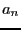be the leading coefficient such that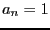. We define the sequence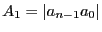,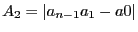,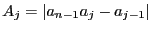. If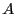is the largest upper bound of the sequence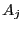, then the modulus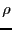of the roots of the polynomial is lower or equal to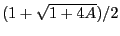.

Jean-Pierre Merlet 2012-12-20